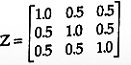# The figure below shows a three phase self-commutated voltage source converter connected to a power system. The converter's dc bus capacitor is marked as C in the figure. The circuit is initially operating in steady state with δ = 0 and the capacitor dc voltage is equal to Vdc0. You may neglect all losses and harmonics. What action should be taken to increase the capacitor dc voltage slowly to a new steady state value

1.  Make δ positive and maintain it at a positive value

2.  Make δ positive and return it to its original value

3.  Make δ negative and maintain it at a negative value

4.  Make δ negative and return it to its original value

4

Make δ negative and return it to its original value

Explanation :
No Explanation available for this question

# The total reactance and total susceptance of a lossless overhead EHV line, operating at 50 Hz, are given by 0.045 pu and 1.2 pu respectively. If the velocity of wave propagation is 3 x 105 km/s, then the approximate length of the line is

1.  122 km

2.  172 km

3.  222 km

4.  272 km

4

172 km

Explanation :
No Explanation available for this question

# Consider the protection system shown in the figure below. The circuit breakers, numbered from 1 to 7 are of identical type. A single line to ground fault with zero fault impedance occurs at the midpoint of the line (at point F), but circuit breaker 4 fails to operate ("stuck breaker"). If the relays are coordinated correctly, a valid sequence of circuit breaker operations is

1.  1, 2, 6, 7, 3, 5

2.  1, 2, 5, 6, 7, 3

3.  5, 6, 7, 3, 1, 2

4.  5, 1, 2, 3, 6, 7

4

5, 6, 7, 3, 1, 2

Explanation :
No Explanation available for this question

# A three phase balanced star connected voltage source with frequency ω rad/ s is connected to a star connected balanced load which is purely inductive. The instantaneous line currents and phase to neutral voltages are denoted by (ia, ib, ic) and (van, vbn, vcn) respectively and their rms values are denoted by V and 1. If R = [van vbn vcn]  , then the magnitude of R is

1.  3 VI

2.  VI

3.  0.7 VI

4.  0

4

3 VI

Explanation :
No Explanation available for this question

# Consider a synchronous generator connected to an infinite bus by two identical parallel transmission lines. The transient reactance x of the generator is 0.1 pu and the mechanical power input to it is constant at 1.0 pu. Due to some previous disturbance, the rotor angle (δ) is undergoing an undamped oscillation, with the maximum value of δ(t) equal to 130°. One of the parallel lines trips due to relay maloperation at an instant when δ(t) = 130° as shown in the figure. The maximum value of the per unit line reactance, x, such that the system does not lose synchronism subsequent to this tripping is

1.  0.87

2.  0.74

3.  0.67

4.  0.54

4

0.67

Explanation :
No Explanation available for this question

# Suppose we define a sequence transformation between "a-b-c" and "p-n-o" variables as follows: where α = ej(2π/3) and k is constant. Now, if it is given that:then,

1.

2.

3.

4.

4Explanation :
No Explanation available for this question

# Consider the two power systems shown in figure A below, which are initially not interconnected, and are operating in steady state at the same frequency. Separate load flow solutions are computed individually for the two systems, corresponding to this scenario. The bus voltage phasors so obtained are indicated on figure A. These two isolated systems are now interconnected by a short transmission line as shown in figure B, and it is found that P1 = P2 = Q1 = Q2 = 0: The bus voltage phase angular difference between generator bus X and generator bus Y after the interconnection is

1.  10°

2.  25°

3.  -30°

4.  30°

4

10°

Explanation :
No Explanation available for this question

# The octal equivalent of the HEX number AB.CD is

1.  253.314

2.  253.632

3.  526.314

4.  526.632

4

253.632

Explanation :
No Explanation available for this question

# If x = Re G(jθ ), and y = lm G(jω) then for a ω → 0+, the Nyquist plot for G(s) = 1 /s(s + 1) (s + 2) becomes asymptotic to the line

1.  x = 0

2.  x = - 3/4

3.  x = y - 1/6

4.  x = y/√3

4

x = - 3/4

Explanation :
No Explanation available for this question

# The system 900/s(s + 1) (s + 9) is to be compensated such that its gain-crossover frequency becomes same as its uncompensated phase-crossover frequency and provides a 45° phase margin. To achieve this, one may use

1.  a lag compensator that provides an attenuation of 20 dB and a phase lag of 45° at the frequency of 3√3 rad /s

2.  a lead compensator that provides an amplification of 20 dB and a phase lead of 45° at the frequency of 3 rad/s

3.  a lag-lead compensator that provides an amplification of 20 dB and a phase lag of 45° at the frequency of √3 rad/

4.  a lag-lead compensator that provides an attenuation of 20 dB and phase lead of 45° at the frequency of 3 rad/s

4